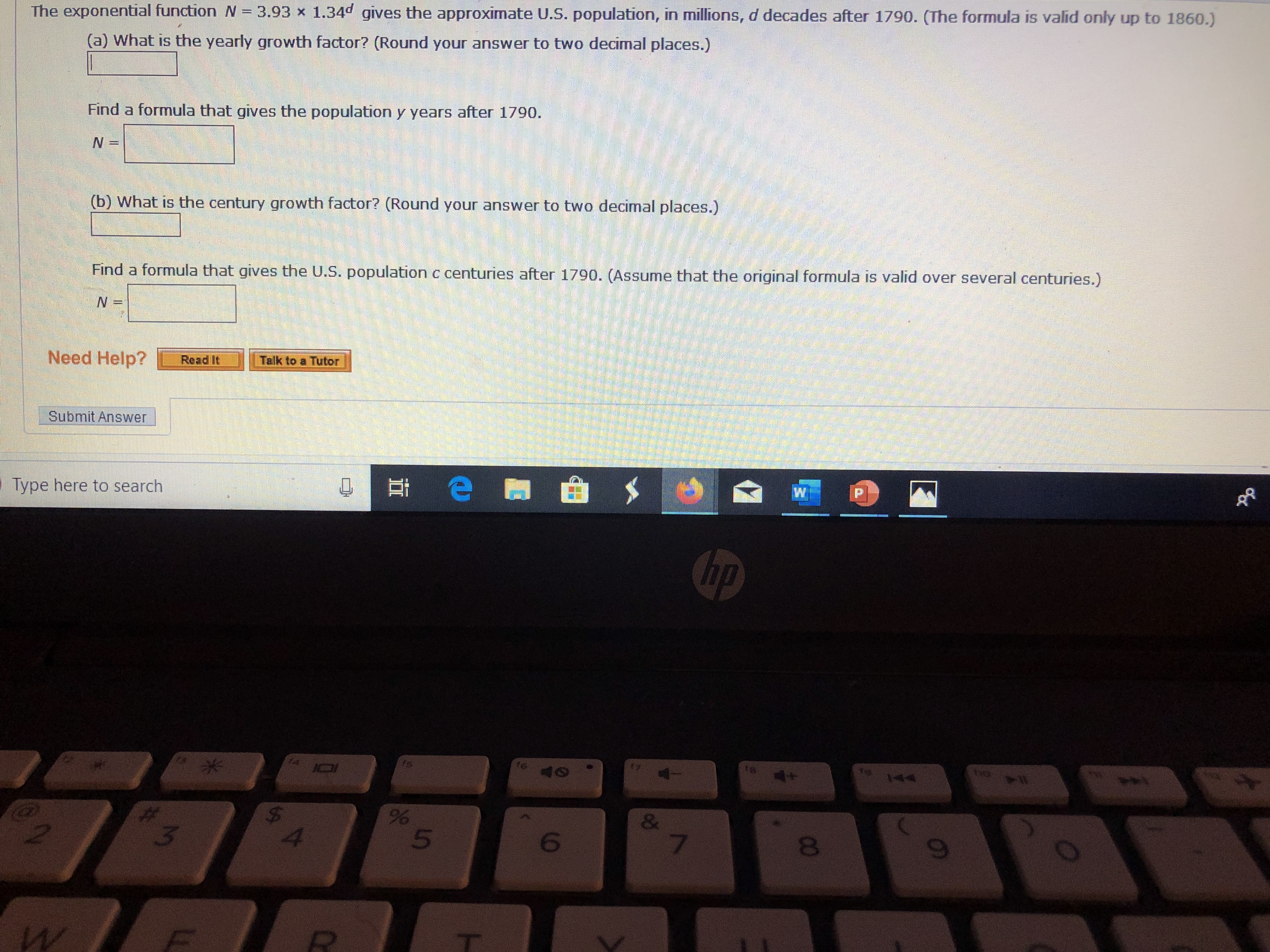# The exponential function N = 3.93 x 1.34d gives the approximate U.S. population, in millions, d decades after 1790. (The formula is valid only up to 1860.) (a) What is the yearly growth factor? (Round your answer to two decimal places.) Find a formula that gives the population y years after 1790. N = (b) What is the century growth factor? (Round your answer to two decimal places.) Find a formula that gives the U.S. population c centuries after 1790. (Assume that the original formula is valid over several centuries.) Talk to a Tutor Need Help? Read It Submit Answer P Type here to search hp t te 144 7 te 40 & % 8 3 2 F CO

Question

what are the blankshelp_outlineImage TranscriptioncloseThe exponential function N = 3.93 x 1.34d gives the approximate U.S. population, in millions, d decades after 1790. (The formula is valid only up to 1860.) (a) What is the yearly growth factor? (Round your answer to two decimal places.) Find a formula that gives the population y years after 1790. N = (b) What is the century growth factor? (Round your answer to two decimal places.) Find a formula that gives the U.S. population c centuries after 1790. (Assume that the original formula is valid over several centuries.) Talk to a Tutor Need Help? Read It Submit Answer P Type here to search hp t te 144 7 te 40 & % 8 3 2 F CO fullscreen

## Expert Answer

### Want to see this answer and more?

Experts are waiting 24/7 to provide step-by-step solutions in as fast as 30 minutes!*

*Response times vary by subject and question complexity. Median response time is 34 minutes and may be longer for new subjects.
Tagged in
MathAlgebra

### Exponents© 2021 bartleby. All Rights Reserved.